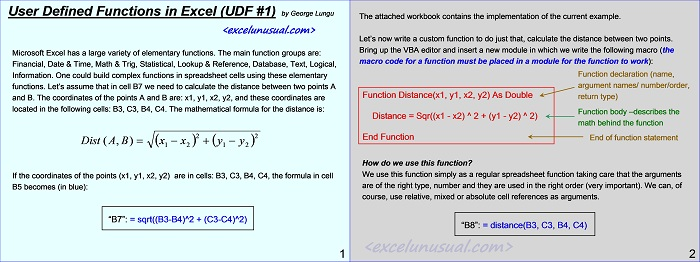# Writing Excel User Defined Functions in VBA – part #1 – the distance function

There are many available built-in spreadsheet functions in Excel. Occasionally one might need a very special custom function.

This short tutorial is an introduction about how to write a user defined function which calculate the distance between two points in a Cartesian plane. The topic will be continued and custom functions will occasionally be used in future models.

We’d love to hear from you.[sociallocker][/sociallocker]

## User Defined Functions in Excel (UDF #1)

by George Lungu

### Introduction:

Microsoft Excel has a large variety of elementary functions. The main function groups are:
Financial, Date & Time, Math & Trig, Statistical, Lookup & Reference, Database, Text, Logical,
Information.

One could build complex functions in spreadsheet cells using these elementary
functions.

Let’s assume that in cell B7 we need to calculate the distance between two points A
and B. The coordinates of the points A and B are: x1, y1, x2, y2, and these coordinates are
located in the following cells: B3, C3, B4, C4. The mathematical formula for the distance is:

Dist( A,B)= sqrt( (x1-x2)^2+(y1-y2)^2+))

<www.excelunusual.com> 1

### Excel implementation:

If the coordinates of the points (x1, y1, x2, y2) are in cells: B3, C3, B4, C4, the formula in cell B5 becomes (in blue):
“B7”: = sqrt((B3-B4)^2 + (C3-C4)^2)

The attached workbook contains the implementation of the current example.

#### Let’s now write a custom function to do just that, calculate the distance between two points.

Bring up the VBA editor and insert a new module in which we write the following macro (the macro code for a function must be placed in a module for the function to work):

Function Distance(x1, y1, x2, y2) As Double          ‘Function declaration (name, argument names/ number/order, return type)

Distance = Sqr((x1 – x2) ^ 2 + (y1 – y2) ^ 2)          ‘ Function body -describes the math behind the function

End Function                                                                ‘End of function statement

#### How do we use this function?

We use this function simply as a regular spreadsheet function taking care that the arguments
are of the right type, number and they are used in the right order (very important). We can, of
course, use relative, mixed or absolute cell references as arguments.
“B8”: = distance(B3, C3, B4, C4)

by George Lungu <www.excelunusual.com>